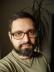## Strong and Weak Primes

Write a script to generate first 10 strong and weak prime numbers.

The two sets of primes are defined with the following formulas:

• p(n) is Strong when `p(n) > [ p(n-1) + p(n+1) ] / 2`
• p(n) is Weak when `p(n) < [ p(n-1) + p(n+1) ] / 2`

It took me some time to realise there is a third set of primes which isn’t mentioned in the above list: there are also Balanced primes. The comparison operator for them is `=`.

To generate primes, I reused the (slightly modified) module I created for the Challenge 012. It caches the primes found so far and extends their list when needed.

``````package My::Primes;
use warnings;
use strict;

sub new { bless [2, 3], shift }

sub is_prime {
my (\$self, \$n) = @_;

\$self->grow until \$self->[-1] >= \$n;

return !! grep \$_ == \$n, @\$self
}

sub grow {
my (\$self) = @_;
my \$candidate = \$self->[-1];
my \$is = 0;
PRIME:
until (\$is) {
\$candidate += 2;
my \$i = 0;
\$is = 1;
while (\$self->[\$i] <= sqrt \$candidate) {
\$is = 0, next PRIME if 0 == \$candidate % \$self->[\$i++];
}
}
push @\$self, \$candidate;
}``````

We can let the module generate primes, what’s left to us is to categorise them as weak, strong, or balanced. Instead of an if-elsif-else or a nested ternary operator, I used a trick: I kept a list of the arrays to push to and used the comparison operator `<=>` to select the particular array. The operator returns -1, 0, or 1, we can easily use these numbers as list subscripts—just keep in mind that -1 comes last.

``````#! /usr/bin/perl
use warnings;
use strict;

my \$p = 'My::Primes'->new;
my (@strong, @weak, @balanced);
while (@strong < 10 || @weak < 10) {
\$p->grow;
push @{
(\@balanced, \@strong, \@weak)[
\$p->[-2] <=> (\$p->[-3] + \$p->[-1]) / 2 ]
}, \$p->[-2];
}``````

And we can output the results (needs `use feature qw{ say };`):

``````say for "Strong: @strong[0 .. 9]",
"Weak: @weak[0 .. 9]",
"Balanced: @balanced";``````

## Vigenère Cipher

I started with a test, based on the Wikipedia page. I decided to use Object Orientation, the constructor would take the keyword as a parameter.

``````use Test::More tests => 2;
my \$v = 'My::Vigenere'->new('LEMON');
is \$v->encode('ATTACKATDAWN'), 'LXFOPVEFRNHR', 'encode';
is \$v->decode('LXFOPVEFRNHR'), 'ATTACKATDAWN', 'decode';``````

I then proceeded to implement the two methods. They ended up very similar to each other, so I deduplicated the common logic into an internal method: in `encode`, we need to “add” the characters and handle the “overflow” beyond Z, in `decode`, we need to “subtract" the characters and handle the “underflow” below A.

``````package My::Vigenere;
use warnings;
use strict;

use constant CHAR_COUNT => 1 + ord('Z') - ord('A');

sub new { bless \\$_, \$_ }
sub encode { \$_->_code(\$_,  1) }
sub decode { \$_->_code(\$_, -1) }

sub _code {
my (\$self, \$string, \$direction) = @_;
my \$x_coded = "";
for my \$i (0 .. length(\$string) - 1) {
my \$ch = substr \$string, \$i, 1;
my \$pch = substr \$\$self, \$i % length \$\$self, 1;
my \$ord = ord(\$ch) + \$direction * (ord(\$pch) - ord('A'));
\$ord -= CHAR_COUNT if \$ord > ord 'Z';
\$ord += CHAR_COUNT if \$ord < ord 'A';
\$x_coded .= chr \$ord ;
}
return \$x_coded
}``````

We need to subtract the `ord('A')` because the character codes don’t start at 0 for A.I blog about Perl.